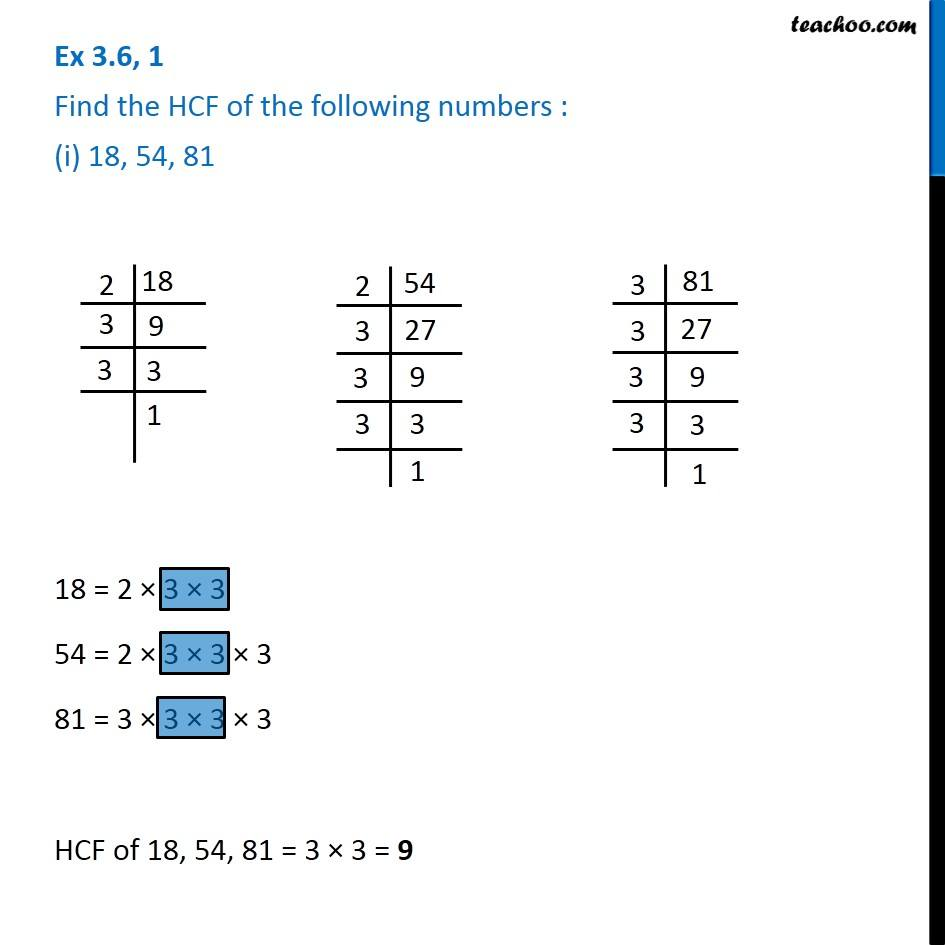1. Chapter 3 Class 6 Playing with Numbers
2. Serial order wise
3. Ex 3.6

Transcript

Ex 3.6, 1 Find the HCF of the following numbers : (i) 18, 54, 81 18 = 2 × 3 × 3 54 = 2 × 3 × 3 × 3 81 = 3 × 3 × 3 × 3 HCF of 18, 54, 81 = 3 × 3 = 9